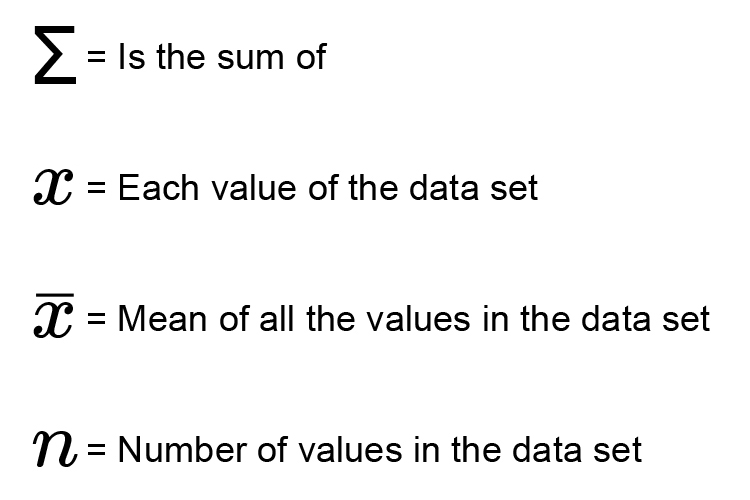# Standard deviation formula

Standard deviation is useful in comparing sets of data which may have the same mean but different ranges. This formula has been developed so that when standard deviation is quoted everyone understands its meaning.

E.g. compare:

i.   15, 15, 15, 14, 16

ii.  2, 7, 14, 22, 30

i.  Mean = (15+15+15+14+16)/5=75/5=15

ii.  Mean = (2+7+14+22+30)/5=75/5=15

Both have a mean of 15 but ii is more spread out.

To show this spread we use the standard deviation formula:

Standard deviation =sigma=sqrt((Sigma(x-bar x)^2)/(n-1))

Where: